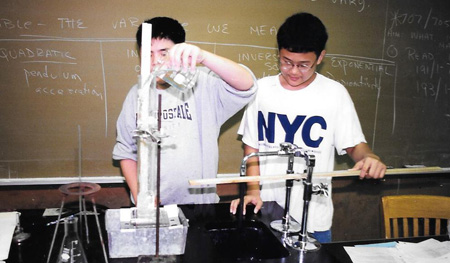# Lab 9: -- Water PressureProblem: What is the relationship between how far water squirts out of a hole in a container and the depth of the hole beneath the water?

Materials: see through milk container and gallon water container with hole, ruler, beakers, large tray.

Hypothesis: Discuss with your what type of relationship you expect to observe in this experiment. Give an explanation for you hypothesis.

Procedure:

1) Put the milk container over onto the metal tray as shown in the figure above.

2) Fill the milk container to the top with water. Take the cork out of the container and let the water empty out.

3) Discuss with your group how the water comes out as the water level decreases.

4) Let the water continue to empty, recording your results with your group.

5) Cork up the hole and fill the milk container again up to a height of 20 cm.

6) Open the cork and measure how far the water squirts out when the height is 20 cm. You will have to continue to add small amounts of water to keep the height of the water at 25 cm.

7) Repeat the above procedure to find out how far water now squirts when the water level is at 20 cm., 15 cm., 10 cm., 1 cm.

8) Complete Table I. in your Results Section.

9) Repeat step 1 7 using the large gallon sized container.

10) Complete Table I. in your Results Section.

Results:

Table I shows how far the water squirted depending on the height of water in the container I.

Height (cm.) Distance water squirts (cm.)

25

20

15

10

5

1

Table II shows how far the water squirted depending on the height of water in the container II.

Height (cm.) Distance water squirts (cm.)

25

20

15

10

5

1

Graph the data from Table I and Table II on the same set of axis.

Discuss the shape of the curve with your group.

Discussion:

1) What type of relationship did you observe? Was your hypothesis correct? Discuss your results.

2) Discuss the reason for the relationship you observe.

3) What are the independent and dependent variables?

4) How are the variables changing with relationship to each other?

5) What happens to the dependent variable when the independent variable increases? decreases?

6) How does the relationship shown in this experiment compare with other relationships you have so far seen?

7) How does the equation for this relationship compare with those of other equations you have studied?

#### Lab Activities and Resources

What is a mathematical relationship andWHAT ARE THE DIFFERENT TYPES OF MATHEMATICAL RELATIONSHIPS that apply to the laboratory exercises in the following activities.

#### Labs

Lab 1:    The Spring Constant  -- Problem:  What is the relationship between how much a spring stretches and the force pulling on the spring?

Lab 2:    The Pendulum  --Problem: What is the relationship between the period of a pendulum and the length of the string of  the pendulum?

Lab 3:   Mass, Volume and Density--   Problem: What is the relationship between the mass of a ball and its volume assuming a constant density?

Lab 4:   Light Intensity--  Problem: What is the relationship between the intensity of a beam of light and the distance from a light source?

Lab 5:   Acceleration--  Problem: What is a the relationship between how the distance travels and the time in travel for an accelerating object?

Lab 6:   Polarization --  Problem:  What is the relationship between how much light passes through a Polaroid filter and the angle the filter is rotated?

Lab  7:   Ohms Law--  Problem:  What is the relationship between current, voltage when there is a constant resistance in an electric circuit.

Lab 8:   Radioactive Decay-- Problem: What is the relationship between the decay of radioactive material and the time allowed for the decay?

Lab 9: Water Pressure-- Problem: What is the relationship between water pressure and depth of water?

Lab 10: Attractive and Repulsive Forces--   Problem: What is the relationship between the distance between two magnets  and the force between them?

Lab 11: Damping Motion--  Problem: What is the relationship between the height a ball bounces and the number of times it has bounced?

Lab 12: Buoyancy - Problem: What is the relationship between the volume of a boat and the weight it can hold?